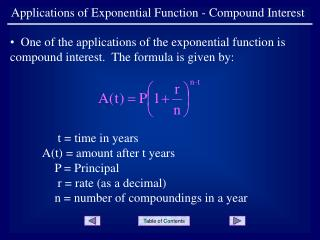DownloadDownload PresentationApplications of Exponential Function - Compound Interest

# Applications of Exponential Function - Compound Interest

Download Presentation## Applications of Exponential Function - Compound Interest

- - - - - - - - - - - - - - - - - - - - - - - - - - - E N D - - - - - - - - - - - - - - - - - - - - - - - - - - -
##### Presentation Transcript

1. Applications of Exponential Function - Compound Interest • One of the applications of the exponential function is compound interest. The formula is given by: t = time in years A(t) = amount after t years P = Principal r = rate (as a decimal) n = number of compoundings in a year

2. Applications of Exponential Function - Compound Interest • Example 1: Find the amount in an account after 4 years, if the initial investment was \$5000, at a rate of 4.5%, compounded monthly. t = 4, P = 5000, r = .045, and n =12

3. Applications of Exponential Function - Compound Interest • Entering the expression into a calculator ... yields an amount of \$5984.07 in the account after 4 years.

4. Applications of Exponential Function - Compound Interest • Example 2: Find the rate of an account with an initial investment of \$10,000 that will yield approximately \$22,477.37 after 12 years, compounded daily. t = 12, A(12) = 22477.37, P = 10,000, and n = 360. (Banking uses 360 rather than 365.)

5. Applications of Exponential Function - Compound Interest • Divide by 10000 ...

6. Applications of Exponential Function - Compound Interest • Raise both sides to a power of the reciprocal of the exponent ... • Note: by pressing the  symbol, the calculator automatically supplies • ANS^.

7. Applications of Exponential Function - Compound Interest • Subtract 1 and multiply by 360 to find ...

8. Applications of Exponential Function - Compound Interest • In the formula for compound interest, the letter n represents the number of compounding periods in one year. As the number of compounding periods increase without bound, it is said that the compounding is “continuous.” The formula for continuous compounding is given by ... where e = 2.71828 ...

9. Applications of Exponential Function - Compound Interest • Example 3: Find the principal that must be invested now at 5% with continuous compounding in order to have \$10,000 in 7 years. • The amount to be invested is \$7046.88

10. Applications of Exponential Function - Compound Interest END OF PRESENTATION Click to rerun the slideshow.